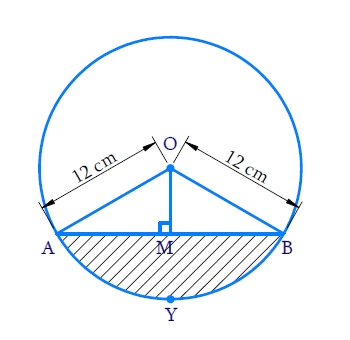# Ex.12.2 Q7 Areas Related to Circles Solution - NCERT Maths Class 10

## Question

A chord of a circle of radius $$12\, \rm{cm}$$subtends an angle of $$120^\circ$$ at the centre. Find the area of the corresponding segment of the circle.

(Use $$\pi =3.14$$ and $$\sqrt{3}=1.73$$)

Video Solution
Areas Related To Circles
Ex 12.2 | Question 7

## Text Solution

What is known?

A chord of a circle with radius $$({r}) = 12 \,\rm{cm}$$ subtends an angle $$(\theta) =120^\circ$$ at the centre.

What is unknown?

Area of segment of the circle.

Reasoning:

In a circle with radius r and angle at the center with degree measure $$\theta$$;

(i) Area of the sector\begin{align} = \frac{{\rm{\theta }}}{{360}^{\rm{o}}} \times \pi {r^2}\end{align}

(ii) Area of the segment = Area of the sector - Area of the corresponding triangle

Draw a figure to visualize the problemHere,\begin{align}{\rm{Radius,}}r=12\rm{cm}\,\,\,\,\,\,{\rm{\theta }} = {120^{\rm{\circ}}}\end{align}

Visually it's clear from that figure that;

AB is the chord subtends$${120^{\rm{\circ}}}$$ angle at the centre.

To find area of the segment AYB, We have to find area of the sector OAYB and area of the$$\Delta AOB$$

(i) Area of sector OAYB \begin{align}= \frac{{\rm{\theta }}}{{{{360}^\circ }}}{\rm{ \times }}\pi {r^2}\end{align}

(ii) Area of  \begin{align}\Delta {\rm{AOB}} = \frac{1}{2} \times \rm{}base \times \rm{}height\end{align}

For finding area of$$\Delta {AOB,}$$draw $${OM}\, \bot\, {AB}$$then find $${AB}$$ and height$${OM.}$$

\begin{align}\Delta {{OMA}}\,& = \,\Delta {{OMB = 9}}{{{0}}^ \circ }\end{align}

$$\therefore \;\Delta \,{{OAM}}\, \cong \Delta \, {OBM}$$(By RHS Congruency)

$$\Rightarrow \quad \angle {{AOM}}= \angle {{BOM}}$$\begin{align} &= \frac{1}{2}\angle {{AOB}}\\& = \frac{1}{2} \times {120^ \circ }\\ &= {60^ \circ }\end{align}

\begin{align}\frac{AM}{OA}=\sin {{60}^\circ } = \frac{\sqrt 3 }{2}\end{align} \begin{align}& ={ AM = \frac{{OA\sqrt 3 }}{2}}\\ &= \frac{{12\sqrt 3 }}{2}\\ &= 6\sqrt 3 \end{align}

\begin{align}{\frac{{OM}}{{OA}}} {= \cos {{60}^\circ } = \frac{1}{2}}\end{align} \begin{align}OM& = \frac{{12\,\rm{{cm}}}}{2}\\&= 6\,\rm{{cm}}\end{align}

$$\text{Area of}\Delta OAB$$\begin{align}&=\frac{{{1}}}{{{2}}}\,\times \,{{base}}\,\times\,{{height}}\\&=\,\frac{{{1}}}{{{2}}}\times\,{{AB}}\, \times \,{{OM}}\end{align}

Steps:Here,\begin{align}{\rm{Radius, }}r = 12\,cm\,\,\,\,\,\,{\rm{\theta }} = {120^{\rm{\circ}}}\end{align}

$$\text{Area of the sector}$$\begin{align}&= \frac{{{{120}^\circ }}}{{{{360}^\circ }}} \times \pi {r^2}\\ &= \frac{1}{3} \times 3.14 \times {(12)^2}\\& = \frac{{452.16}}{6}\,\rm{cm^2}\\ &= 150.72\,\rm {c m^2}\end{align}

Draw a perpendicular $${OM}$$ from $${O}$$ to chord $${AB}$$

In $$\Delta \,{AOM}$$and $$\Delta \,{BOM}$$

$${AO}={BO}$$(radii of circle)

$${OM} = {OM}$$(common)

$$\angle {OMA} = \angle {OMB = {{90}^\circ}}$$

$$\therefore \;\Delta {AOM} \cong \Delta {BOM}$$(By RHS Congruency)

\begin{align}\therefore \angle {AOM} &= \angle {BOM} \\&= \frac{1}{2} \angle {AOB} = 60^\circ \end{align}

In $$\Delta {AOM}$$

\begin{align}\frac{{{{AM}}}}{{{{OA}}}}\, &=\,{{sin}}\,{{6}}{{{0}}^{{o}}}\\{{AM}}\, &= \,{{OA}}\frac{{\sqrt {{3}} }}{{{2}}}\\&=\frac{{{{12}}\sqrt {{3}} }}{{{2}}}\\&= 6\sqrt {{3}}\,\rm{{cm}}\\{AM} &= {2AM}\\&= 12\sqrt {{3}} \,\rm{{cm}}\end{align}

Area of $$\Delta \,{OAB}$$

\begin{align}&=\frac{1}{2}\times AB\times OM \\&=\frac{1}{2}\times 12\sqrt{3}\times 6 \\&=36\sqrt{3} \\ &=36\times 1.73 \\&=62.28\,\rm{c}{{{m}}^{2}} \\\end{align}

Area segment $${APB} =$$Area of sector $${OAPB}$$$$-$$Area of$$\Delta \,{OAB}\,$$

\begin{align}&=150.72-62.28\\&=88.44\; \rm{cm^2} \end{align}

Learn from the best math teachers and top your exams

• Live one on one classroom and doubt clearing
• Practice worksheets in and after class for conceptual clarity
• Personalized curriculum to keep up with school